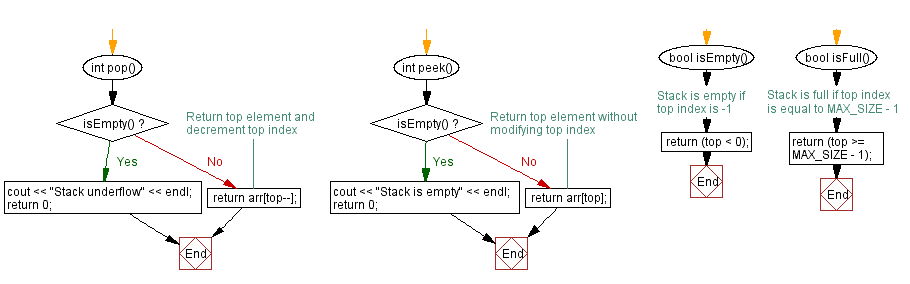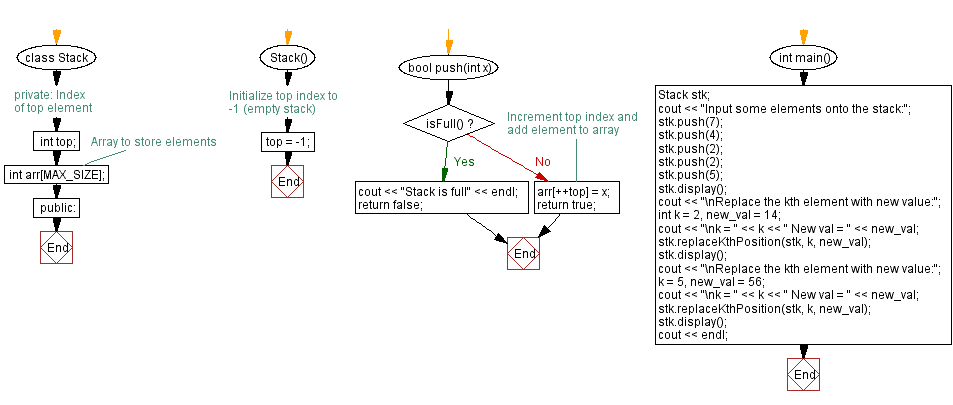﻿ C++ Replace the kth element with new value in a stack

# C++ Stack Exercises: Replace the kth element with new value in a stack

## C++ Stack: Exercise-15 with Solution

Write a C++ program to replace the kth element with new value in a stack (using an array).

Test Data:
Input some elements onto the stack:
Stack elements are: 5 2 2 4 7
Replace the kth element with new value:
k = 2 New val = 14

Sample Solution:

C++ Code:

``````#include <iostream>

using namespace std;
#define MAX_SIZE 15 // Maximum size of stack

class Stack {
private:
int top; // Index of top element
int arr[MAX_SIZE]; // Array to store elements
public:
Stack() {
top = -1; // Initialize top index to -1 (empty stack)
}

bool push(int x) {
if (isFull()) {
cout << "Stack is full" << endl;
return false;
}
// Increment top index and add element to array
arr[++top] = x;
return true;
}
int pop() {
if (isEmpty()) {
cout << "Stack underflow" << endl;
return 0;
}
return arr[top--];
}

int peek() {
if (isEmpty()) {
cout << "Stack is empty" << endl;
return 0;
}
return arr[top];
}

bool isEmpty() {
// Stack is empty if top index is -1
return (top < 0);
}

bool isFull() {
// Stack is full if top index is equal to MAX_SIZE - 1
return (top >= MAX_SIZE - 1);
}

void display() {
if (top < 0) {
cout << "Stack is empty" << endl;
return;
}
cout << "\nStack elements are: ";
for (int i = top; i >= 0; i--)
cout << arr[i] << " ";
cout << endl;
}

void replaceKthPosition(Stack& stk, int k, int value) {
if (stk.isEmpty()) {
cout << "Stack is empty." << endl;
return;
}

int size = stk.top + 1;
int temp[size];
int count = 0;

//The stack elements are copied into a temporary array
while (!stk.isEmpty()) {
temp[count++] = stk.pop();
}

// Replace the kth element in the temporary array
if (k > count || k <= 0) {
cout << "Invalid position." << endl;
return;
} else {
temp[k-1] = value;
}

// The elements are pushed back into the stack in reverse order
for (int i = count-1; i >= 0; i--) {
stk.push(temp[i]);
}
}

};
int main() {
Stack stk;
cout << "Input some elements onto the stack:";
stk.push(7);
stk.push(4);
stk.push(2);
stk.push(2);
stk.push(5);
stk.display();
cout << "\nReplace the kth element with new value:";
int  k = 2, new_val = 14;
cout << "\nk = " << k << " New val = " << new_val;
stk.replaceKthPosition(stk, k, new_val);
stk.display();
cout << "\nReplace the kth element with new value:";
k = 5, new_val = 56;
cout << "\nk = " << k << " New val = " << new_val;
stk.replaceKthPosition(stk, k, new_val);
stk.display();
cout << endl;
}
``````

Sample Output:

```Input some elements onto the stack:
Stack elements are: 5 2 2 4 7

Replace the kth element with new value:
k = 2 New val = 14
Stack elements are: 5 14 2 4 7

Replace the kth element with new value:
k = 5 New val = 56
Stack elements are: 5 14 2 4 56
```

Flowchart:cpp-stack-exercise-flowchart-15-1

CPP Code Editor: